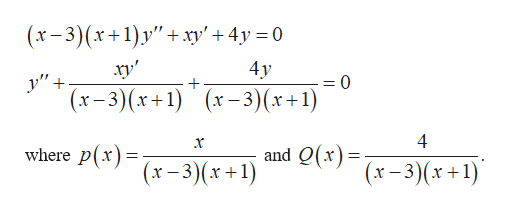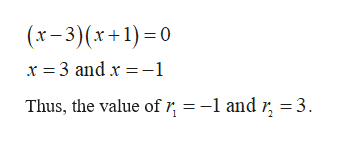Determine a lower bound for the radius of convergence of series solutions about the given point x0 for the differential equation:(x2-2x-3)y"+xy'+4y=0x0=4x0=0

Question

Determine a lower bound for the radius of convergence of series solutions about the given point x0 for the differential equation:

(x2-2x-3)y"+xy'+4y=0

• x0=4
• x0=0
Step 1

Given:

The differential equation is (x2-2x-3)y”+xy’+4y=0

Step 2

Calculation:

Rewrite the given equation as follows.help_outlineImage Transcriptionclose(х-3)(х+1)у"+ ху' + 4у 30 4у ху" -0 у" + (х-3)(x+1) (х-3)(*+1) 4 and Q(*) - (x-3)(х+1) х where P (x-3)(x +1) fullscreen
Step 3

The lower bound of the given function is the minimum distance from r1, r2 and x0, where r1 and r2 are the zeros of the given ...help_outlineImage Transcriptionclosex-3)(x+1)0 x 3 and x 1 = -1 and r, =3 Thus, the value of fullscreen

Want to see the full answer?

See Solution

Want to see this answer and more?

Our solutions are written by experts, many with advanced degrees, and available 24/7

See Solution
Tagged in

Math